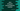# Java ArrayList ensureCapacity method## Java ArrayList ensureCapacity method:

The ensureCapacity method is used to increase the capacity of an ArrayList if required. This method is defined in java.util.ArrayList class. In this post, we will learn how to use this method with examples.

## Definition of ensureCapacity:

The ensureCapacity method is defined as:

``public void ensureCapacity(int minCapacity)``

It takes one parameter, minCapacity. The ArrayList ensures that it will hold at least minCapacity number of elements.

Let’s try this method with an example.

### Example of ensureCapacity:

Let’s take a look at the below example:

``````import java.util.ArrayList;

public class Main {

public static void main(String[] args) {
ArrayList<Integer> numList = new ArrayList<>();
numList.ensureCapacity(40);

System.out.println(numList);
}
}``````

In this example, numList is an ArrayList of integers. Three numbers are added to this ArrayList and the ensureCapacity method is used with 40 as its parameter.

If you run this program, you will see that it will print only the numbers we added to the ArrayList:

``[1, 2, 3]``

### Example of ensureCapacity with a smaller minCapacity than the number of items:

We can add a smaller minCapacity value than the number of items added to the ArrayList:

``````import java.util.ArrayList;

public class Main {

public static void main(String[] args) {
ArrayList<Integer> numList = new ArrayList<>();
numList.ensureCapacity(1);

System.out.println(numList);
}
}``````

In this example, we added three items to the ArrayList and called the ensureCapacity method with 1 as its parameter.

Since minCapacity can define the minimum number of elements an ArrayList can hold, it will not make any difference.

If you run this program, it will print:

``[1, 2, 3]``

### Example of ensureCapacity with a negative value:

We can also provide a negative value to the ensureCapacity method. It will not throw any exceptions:

``````import java.util.ArrayList;

public class Main {

public static void main(String[] args) {
ArrayList<Integer> numList = new ArrayList<>();